RRB NTPC Mock Test - 12 (English)

# RRB NTPC Mock Test - 12 (English)

Test Description

## 100 Questions MCQ Test RRB NTPC Mock Tests Series | RRB NTPC Mock Test - 12 (English)

RRB NTPC Mock Test - 12 (English) for Railways 2022 is part of RRB NTPC Mock Tests Series preparation. The RRB NTPC Mock Test - 12 (English) questions and answers have been prepared according to the Railways exam syllabus.The RRB NTPC Mock Test - 12 (English) MCQs are made for Railways 2022 Exam. Find important definitions, questions, notes, meanings, examples, exercises, MCQs and online tests for RRB NTPC Mock Test - 12 (English) below.
Solutions of RRB NTPC Mock Test - 12 (English) questions in English are available as part of our RRB NTPC Mock Tests Series for Railways & RRB NTPC Mock Test - 12 (English) solutions in Hindi for RRB NTPC Mock Tests Series course. Download more important topics, notes, lectures and mock test series for Railways Exam by signing up for free. Attempt RRB NTPC Mock Test - 12 (English) | 100 questions in 90 minutes | Mock test for Railways preparation | Free important questions MCQ to study RRB NTPC Mock Tests Series for Railways Exam | Download free PDF with solutions
 1 Crore+ students have signed up on EduRev. Have you?
RRB NTPC Mock Test - 12 (English) - Question 1

### A photocell converts light energy into ________.

Detailed Solution for RRB NTPC Mock Test - 12 (English) - Question 1
• A photocell converts light energy into electrical energy. There are mainly three kinds of photocells, which are photovoltaic, photoconductive, and photo emissive.
• The Photoelectric effect was explained by Albert Einstein.
RRB NTPC Mock Test - 12 (English) - Question 2

### How many articles were there originally in the Constitution of India?

Detailed Solution for RRB NTPC Mock Test - 12 (English) - Question 2
• There were 395 articles originally in the Constitution of India.
• Presently 448 articles are there in Constitution.
• Indian Constitution was originally written in English.
• Prem Behari Narain Raizada wrote the Indian constitution by hand.
• Dr. BR Ambedkar is known as the father of the Indian Constitution.
• Indian Constitution was adopted by the Constituent Assembly of India on 26 November 1949 and became effective on 26 January 1950.
RRB NTPC Mock Test - 12 (English) - Question 3

### There are six persons Saurav, Harshita, Jay, Ashwini, Jagannath and Sakshi, who got different marks in the examination. Ashwini got more marks than all of them. Jay got more marks than two of them. Jagannath got less marks than only Ashwini. Saurav got less marks than 5 persons. Harshita got more marks than Jay while Sakshi got less marks than Jay. How many persons scored more marks than Sakshi?

Detailed Solution for RRB NTPC Mock Test - 12 (English) - Question 3

1) Ashwini got more marks than all of them. Jay got more marks than two of them.

Ashwini > ……. > ……. > Jay > …… > …….

2) Jagannath got less marks than only Ashwini.

Ashwini > Jagannath > ……. > Jay > …… > …….

3) Saurav got less marks than 5 persons.

(Therefore Saurav got the least marks)

Ashwini > Jagannath > ……. > Jay > …… > Saurav

4) Harshita got more marks than Jay while Sakshi got less marks than Jay.

Ashwini > Jagannath > Harshita > Jay > Sakshi > Saurav

Hence, four people scored more than Sakshi.

RRB NTPC Mock Test - 12 (English) - Question 4

ISRO’s Mars Orbiter Mission was launched using which of the following launch vehicle?

Detailed Solution for RRB NTPC Mock Test - 12 (English) - Question 4
• The Mars Orbiter Mission was launched from the Satish Dhawan Space Centre Andhra Pradesh, using a Polar Satellite Launch Vehicle (PSLV) C25 XL on 5 November 2013.
• It is one of the world's most reliable launch vehicles.
• The XL variant was earlier used to launch Chandrayaan (2008), GSAT-12 (2011) and RISAT-1 (2012).
• MOM is India's first venture into the interplanetary space.
• It will explore and observe Mars surface features, morphology, mineralogy and the Martian atmosphere.
RRB NTPC Mock Test - 12 (English) - Question 5

The third proportional to (x2 – y2) and (x – y) is:

Detailed Solution for RRB NTPC Mock Test - 12 (English) - Question 5

Let the third proportion be a

(x2 – y2) : (x – y) :: (x – y) : a

a = (x – y)2 /(x2 – y2)= (x – y)/(x + y)

RRB NTPC Mock Test - 12 (English) - Question 6

In ΔABC, measure of ∠C is 90°. If sin B = 24/25 and BC = 3.5 cm, then what is the length (in cm) of side AB?

Detailed Solution for RRB NTPC Mock Test - 12 (English) - Question 6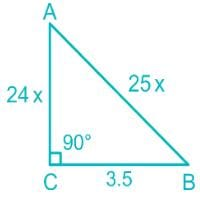If sin B = 24/25

sin B = Perpendicular/Hypotenuse = AC/AB = 24/25

Taking AC = 24 * x ,  AB = 25 * x and BC=3.5 cm

By the Pythagoras theorem, x = 0.5

⇒ AC =24 * x =12 cm

=>AB = 25 * x = 12.5 cm

∴ The length of AB is 12.5 cm

RRB NTPC Mock Test - 12 (English) - Question 7

Dry ice is the solid form of which of the following?

Detailed Solution for RRB NTPC Mock Test - 12 (English) - Question 7

Dry ice is the solid form of Carbon Dioxide. It has lower temperature than the water ice and is used as a cooling agent.

RRB NTPC Mock Test - 12 (English) - Question 8

The World Kidney Day 2019 was observed on which of the following days?

Detailed Solution for RRB NTPC Mock Test - 12 (English) - Question 8
• The World Kidney Day 2019 was observed on March 14, 2019.
• This Day is observed annually on the second Thursday of March.
•  It aims to raise awareness about the importance of our kidneys.
• Theme: ‘Kidney Health for Everyone Everywhere’.
RRB NTPC Mock Test - 12 (English) - Question 9

P, Q and R undertook a work for Rs. 48000. Together P and Q complete 5/12th part of the work. What is the share (in Rs.) of R?

Detailed Solution for RRB NTPC Mock Test - 12 (English) - Question 9

Together P and Q complete 5/12th part of the work

∴ Part of work completed by R

= 1 - (5/12)

= 7/12

The total amount for the work = Rs. 48000

∴ Share of R = (7/12) × 48000

= 7 × 4000

= 28000

RRB NTPC Mock Test - 12 (English) - Question 10

How many triangles are there in the given figure?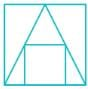Detailed Solution for RRB NTPC Mock Test - 12 (English) - Question 10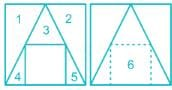Thus there are 6 triangles in the given figure.

RRB NTPC Mock Test - 12 (English) - Question 11

What is the ratio of length and breadth of the Indian national flag?

Detailed Solution for RRB NTPC Mock Test - 12 (English) - Question 11
• The ratio of length and breadth of the Indian national flag is 3 : 2.
• It is a horizontal tricolor of deep saffron (Kesari) at the top, white in the middle and dark green at the bottom in equal proportion.
• It became the official flag of the Dominion of India on 15 August 1947.
• Pingali Venkayya is considered as the designer of the Indian National Flag.
RRB NTPC Mock Test - 12 (English) - Question 12

According to the Union Budget 2019, individuals having an income of up to _______ won’t pay any tax.

Detailed Solution for RRB NTPC Mock Test - 12 (English) - Question 12
• Present Finance Minister - Arun Jaitley
• The Finance Minister during the budget presentation was Piyush Goyal. He proposed that individuals having an income of up to rupees five (5) lakh will have not have to pay any tax.
• Even persons having a gross income up to rupees 6.5 lakh may not be required to pay any income tax if they make investments in Provident Funds and specified savings and insurance.
RRB NTPC Mock Test - 12 (English) - Question 13

Gokak Falls is located on which of the following river?

Detailed Solution for RRB NTPC Mock Test - 12 (English) - Question 13
• The Gokak Falls is located in Belagavi, Karnataka on the Ghataprabha River.
• Gokak gets its name due to the Goki trees found in abundance in these areas.
• Its shape, color, height and speed helped it earn the tag of 'mini Niagara’.
RRB NTPC Mock Test - 12 (English) - Question 14

Which of the following numbers is the largest?

Detailed Solution for RRB NTPC Mock Test - 12 (English) - Question 14

Solving the given options:

(6 + 6 + 6)2 = 18 = 324

{(6 + 6)2}2 =  (122)2 = 20736

(6 × 6 × 6)2 = (216)2 = 46656

6 + 62 + (6)3 = 6 + 36 + 216 = 258

∴ On comparing all the options, (6 × 6 × 6)2 is the largest number.

RRB NTPC Mock Test - 12 (English) - Question 15

Which among the following is the biggest local urban government?

Detailed Solution for RRB NTPC Mock Test - 12 (English) - Question 15
• Municipal Corporation is the biggest local urban government.
• Municipal Council is for the smaller urban area.
• Nagar Panchayat is for areas in transition from rural to urban.
RRB NTPC Mock Test - 12 (English) - Question 16

The average of 6 numbers is 18. If one number is excluded, the average becomes 17. What is the excluded number?

Detailed Solution for RRB NTPC Mock Test - 12 (English) - Question 16

Average = (sum of elements)/number of elements

Given,

The average of 6 numbers is 18.

Sum of 6 numbers = 18 × 6 = 108

Given,

If one number is excluded, the average becomes 17.

Average of 5 numbers = 17

Sum of 5 numbers = 17 × 5 = 85

Excluded number =

= sum of 6 numbers - sum of 5 numbers

= 108 - 85

= 23

∴ The excluded number is 23.

RRB NTPC Mock Test - 12 (English) - Question 17

Wind streams show an ________ diversion in Northern hemisphere due to Coriolis force.

Detailed Solution for RRB NTPC Mock Test - 12 (English) - Question 17
• Due to the Earth's rotation wind movements across the Earth's surface are diverted to the right in the Northern Hemisphere and to the left in the Southern Hemisphere.
• The rotation of the earth about its axis affects the direction of the wind. This force is called the Coriolis force.
• It not only affects wind movement but it influences all of the movements across the Earth's surface.
• This direction is determined by Farrell’s Law.
RRB NTPC Mock Test - 12 (English) - Question 18

As per the interim budget of 2019, a separate department for which of the following will be created?

Detailed Solution for RRB NTPC Mock Test - 12 (English) - Question 18
• As per the interim budget of 2019, a separate department of Fisheries will be created.
• Additionally, 2 percent interest subvention to farmers pursuing animal husbandry and fisheries will also be given.
• NOTE: A separate department for fisheries and a separate commission for cows will be created.
RRB NTPC Mock Test - 12 (English) - Question 19

Arrange the given words in the sequence in which they occur in the dictionary.

i. Faculty
ii. Faith
iii. Faint
iv. Familiar

Detailed Solution for RRB NTPC Mock Test - 12 (English) - Question 19

On arranging given word as per dictionary order,

i. Faculty
iii. Faint
ii. Faith
iv. Familiar

Hence, “I, iii, ii, iv” is correct order as per dictionary.

RRB NTPC Mock Test - 12 (English) - Question 20

In the following question, select the number which can be placed at the sign of question mark (?) from the given alternatives.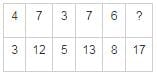Detailed Solution for RRB NTPC Mock Test - 12 (English) - Question 20

Let the elements of matrix be A11, A12, A21, and A22 as shown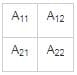Then,

(A11 + A12 + A21) - 2 = A22

In Matrix 1 → 4 + 7 + 3 - 2 = 14 - 2 = 12.

In Matrix 2 → 3 + 7 + 5 - 2 = 15 - 2 = 13.

Similarly,

In Matrix 3 → 6 + ? + 8 - 2 = 17 → ? = 17 - 12 = 5.

Hence, 5 is the correct answer.

RRB NTPC Mock Test - 12 (English) - Question 21

Which of the following is most reactive in nature?

Detailed Solution for RRB NTPC Mock Test - 12 (English) - Question 21
• An atom's reactivity is determined by the number of electrons in its outermost shell.
• Less valence electron means high reactivity.

Valence electron in elements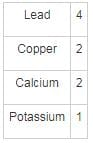RRB NTPC Mock Test - 12 (English) - Question 22

The junction between two nerve cells is known as?

Detailed Solution for RRB NTPC Mock Test - 12 (English) - Question 22
• The junction between two nerve cells is known as the Synapse.
• The information is passed between two nerve cells through synapse in the form of chemical or electrical signals.
• At a synapse, the plasma membrane of the signal-passing neuron comes into the close with the membrane of the target cell.
• A synaptic connection between a neuron and a muscle cell is known as a neuromuscular junction.
RRB NTPC Mock Test - 12 (English) - Question 23

What is the dimensional formula of magnetic flux?

Detailed Solution for RRB NTPC Mock Test - 12 (English) - Question 23

We know,
ϕ = B Δ S cosθ
Here, cosθ is dimensionless
ΔS is the surface area of the surface placed in the magnetic field = [L2]
Bis the magnetic field =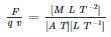So, Dimensional formula of flux =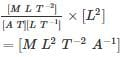RRB NTPC Mock Test - 12 (English) - Question 24

wo lines 2x – 3y = 6 and ax – 9y = 1 are parallel to each other. Find the value of a.

Detailed Solution for RRB NTPC Mock Test - 12 (English) - Question 24

Given line 2x – 3y = 6

We can write y = 2x/3 – 2

∵ Slope of line y = mx + c is equal to m,

⇒ Slope of line = 2/3

Considering other line ax – 9y = 1

We can write, y = a/9 – 1/9

⇒ Slope of line = a/9

∵ Slope of parallel lines is equal

⇒ a/9 = 2/3

⇒ a = 9 × 2/3

∴ a = 6

RRB NTPC Mock Test - 12 (English) - Question 25

Which among the following is the result of the excess of Phosphorus?

Detailed Solution for RRB NTPC Mock Test - 12 (English) - Question 25
• Kidney Failure is a result of the excess of Phosphorus.
• It is important for the structure of DNA, a transporter of energy (ATP), a component of the cellular membrane, helps strengthen bones.
• Deficiency of Phosphorus can cause hypophosphatemia.
RRB NTPC Mock Test - 12 (English) - Question 26

Correct the following equation by interchanging the two signs and two numbers (taking one set of the first elements from the left)
52 × 4 ÷ 2 + 6 × 10 ÷ 3 = 31

Detailed Solution for RRB NTPC Mock Test - 12 (English) - Question 26

Given equation is 52 × 4 ÷ 2 + 6 × 10 ÷ 3 = 31

Let’s check each of the option,

1. Interchanging 4, 2 and ×, ÷

Equation will become, 52 ÷ 2 × 4 + 6 × 10 ÷ 3 = 124 ≠ 31

2. Interchanging 2, 6 and ÷, +

Equation will become, 52 × 4 + 6 ÷ 2 × 10 ÷ 3 = 218 ≠ 31

3. Interchanging 6, 3 and ×, +

Equation will become, 52 + 4 ÷ 2 + 3 × 10 ÷ 6 = 59 ≠ 31

4. Interchanging 10, 6 and ×, ÷

Equation will become, 52 ÷ 4 × 2 + 10 ÷ 6 × 3 = 31

Hence, by interchanging 10, 6 and ×, ÷ we get correct answer.

RRB NTPC Mock Test - 12 (English) - Question 27

Primary education is most essential and forms the base of an individual. Education is very important to lead a good life.
Which of the following is a statement that can be inferred from the facts stated in the above statement?

Detailed Solution for RRB NTPC Mock Test - 12 (English) - Question 27

Here the fact-Education is important to lead a good life

Assumption-If primary education is not properly attained, base of an individual may not be strongly built.

From both the statements we can infer that education is the soul of one’s life. This is exactly what is told in option 4. Rest all options are also valid but cannot be logically inferred from the given statements.

RRB NTPC Mock Test - 12 (English) - Question 28

Average age of 6 boys is 14 years. Average age of 11 girls is 12 years. What is the average age (in years) of all boys and girls?

Detailed Solution for RRB NTPC Mock Test - 12 (English) - Question 28

Average of 6 boys = Sum of age of 6 boys/6

⇒ 14 = sum of ages of 6 boys/6

⇒ Sum of age of 6 boys = 84

Now,

Average of 11 girls = sum of age of 11 girls/11

⇒ 12 = sum of age of 11 girls/11

⇒ Sum of age of 11 girls = 132

Now,

Sum of age of all girls and boys together = 84 + 132 = 216

∴ Average = 216/17 = 12.7

RRB NTPC Mock Test - 12 (English) - Question 29

Which among the following disease is caused due to deficiency of vitamin D?

Detailed Solution for RRB NTPC Mock Test - 12 (English) - Question 29
• Osteomalacia is a disease caused due to the deficiency of Vitamin D.
• Arthritis is a disease caused due to the deficiency of Synovial Fluid.
• Gout is a disease caused due to the accumulation of Uric Acid.
• Pellagra is a disease caused due to deficiency of Vitamin B3
RRB NTPC Mock Test - 12 (English) - Question 30

If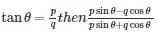is equal to:

Detailed Solution for RRB NTPC Mock Test - 12 (English) - Question 30

Given,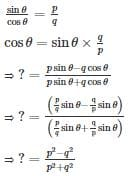RRB NTPC Mock Test - 12 (English) - Question 31

In the following figure, square represents pharmacists, triangle represents Dancers, circle represents Gynecologists and rectangle represents Women. Which set of letters represents Gynecologists who are neither Women nor Dancers?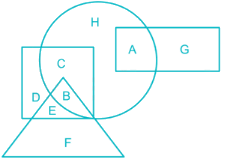Detailed Solution for RRB NTPC Mock Test - 12 (English) - Question 31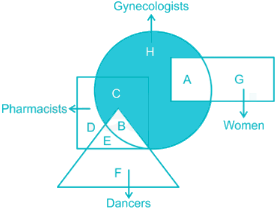Gynecologists who are neither Women nor Dancers are represented by ‘C and H’.

RRB NTPC Mock Test - 12 (English) - Question 32

If p and q are in the ratio 4 : 3 and their LCM is 36, find p + q =?

Detailed Solution for RRB NTPC Mock Test - 12 (English) - Question 32

Let the numbers p and q be 4x and 3x respectively.

4x = 4 × x

3x = 3 × x

LCM (4x, 3x) = 4 × 3 × x = 36

∴ x = 36/12 = 3

The numbers p and q are 12 and 9 respectively.

∴ Their sum = 12 + 9 = 21

RRB NTPC Mock Test - 12 (English) - Question 33

There would be 15% loss if a toy is sold for Rs 25.50. At what price should it be sold to earn a profit of 25%

Detailed Solution for RRB NTPC Mock Test - 12 (English) - Question 33

Given,

The selling price of toy = 25.5

SP = CP - (CP × loss%)

⇒ 25.5 = 0.85 CP

⇒ CP = 30

To get a profit of 25%, he should sell it for,

SP = CP + (CP × profit%)

⇒ SP = 30 + 30 × 25/100 = 75/2 = 37.5

RRB NTPC Mock Test - 12 (English) - Question 34

Select the correct combination of mathematical signs to replace ∗ signs and to balance the given equation.
19 ∗ 20 ∗ 6 ∗ 33

Detailed Solution for RRB NTPC Mock Test - 12 (English) - Question 34

Let us check out,

1) 19 - 20 + 6 = 33, False

2) 19 + 20 - 6 = 33, True

3) 19 = 20 + 6 - 33, False

4) 19 = 20 - 6 + 33, False

Hence, ‘+, -, =’ is the correct sequence

RRB NTPC Mock Test - 12 (English) - Question 35

In a classroom there are certain number of benches. If 6 students are made to sit on a bench, then to accommodate all of them, one more bench is needed. However, if 7 students are made to sit on a bench, then after accommodating all of them, space for 5 students is left. What is the total number of students in the class?

Detailed Solution for RRB NTPC Mock Test - 12 (English) - Question 35

Let the total number of students in class be N.

No. of benches be x.

Given,

⇒ N = 6x + 6

⇒ N = 7x - 5

Then,

⇒ 6x + 6 = 7x - 5

⇒ x = 11

∴ Total number of Students = 6 × 11 + 6 = 72

RRB NTPC Mock Test - 12 (English) - Question 36

The difference between 81% and 49% of a number is 768. What is the 48% of the number?

Detailed Solution for RRB NTPC Mock Test - 12 (English) - Question 36

Let the number be x

81% of x = 0.81x

49% of x = 0.49x

Difference between 81% of x and 49% of x = 768

⇒ 0.81x - 0.49x = 768

⇒ 0.32x = 768

⇒ x = 2400

48% of x = 0.48x = 0.48 × 2400 = 1152

RRB NTPC Mock Test - 12 (English) - Question 37

A sum of Rs. 4000 amounts to Rs. 5200 in 10 yr by simple interest.What will be the total interest if the rate of interest is increased by 4%?

Detailed Solution for RRB NTPC Mock Test - 12 (English) - Question 37

S.I = PRT/100

Here P = Principle, R = Rate T = Time, S.I = Simple interest

S. I = 5200 – 4000 = (4000 × R × 10)/100

⇒ R = (1200 × 100)/(4000 × 10) = 3%

New rate = 3 + 4 = 7%

Interest = (4000 × 7 × 10)/100 = Rs. 2800

RRB NTPC Mock Test - 12 (English) - Question 38

Which one of the following statements is not true about Gamma radiations?

Detailed Solution for RRB NTPC Mock Test - 12 (English) - Question 38
• Gamma emission neither changes the atomic number nor the atomic mass.
• Gamma rays are high-energy photons with a very short wavelength.
• They have high penetrating power as compared to alpha and beta radiations.
• They arise from the radioactive decay of atomic nuclei.
RRB NTPC Mock Test - 12 (English) - Question 39

In the following question, select the number which can be placed at the sign of question mark (?) from the given alternatives.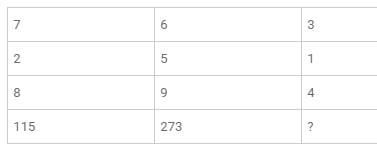Detailed Solution for RRB NTPC Mock Test - 12 (English) - Question 39

The pattern is:
7 x 2 x 8 + 3  = 112 + 3 = 115
6 x 5 x 9 + 3 = 270 + 3 = 273
Similarly,
3 x 1 x 4 + 3 = 12 + 3  = 15

RRB NTPC Mock Test - 12 (English) - Question 40

In which of the following year the Department of Scientific and Industrial Research was set up?

Detailed Solution for RRB NTPC Mock Test - 12 (English) - Question 40
• The Department of Scientific and Industrial Research was set up in 1985.
• It is a part of the Ministry of Science and Technology.
• The Minister for Ministry of Science & Technology is Dr. Harsh Vardhan.
RRB NTPC Mock Test - 12 (English) - Question 41

What will come in place of question mark (?) in the following question?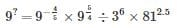Detailed Solution for RRB NTPC Mock Test - 12 (English) - Question 41

Given,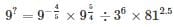Solving RHS,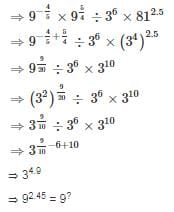RRB NTPC Mock Test - 12 (English) - Question 42

If Standard deviation of a data set is 13. What is the variance?

Detailed Solution for RRB NTPC Mock Test - 12 (English) - Question 42

We know,

S.D =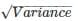= Variance = (S. D)2

= (13)2

= 169

RRB NTPC Mock Test - 12 (English) - Question 43

A and B together do a job in 15 days and A alone could do the same job in 20 days. How many days would B take to do half the job if he worked alone?

Detailed Solution for RRB NTPC Mock Test - 12 (English) - Question 43

Work done by A in 1 day can be given as 1/20th of the total work

Let the work done by B in 1 day be y of the total work

From the problem statement

⇒ 15 × (1/20 + y) = 1

⇒ y = 1/15 - 1/20 = 1/60

Time required by B to complete the work is 60 days

Time required to complete the half work is ½ × 60 = 30 days

∴ The time required to complete half of the work is 30 days

RRB NTPC Mock Test - 12 (English) - Question 44

In which year the Sirima-Shastri Pact was signed?

Detailed Solution for RRB NTPC Mock Test - 12 (English) - Question 44
• The Sirima-Shastri Pact was signed on 30 October 1964.
• It was an agreement that was signed between Sirimavo Bandaranaike, the Prime Minister of Sri Lanka, and Lal Bahadur Shastri, the Prime Minister of India.
• It was an agreement determining the future of people of Indian origin in Ceylon.
RRB NTPC Mock Test - 12 (English) - Question 45

Which among the following cities is known as Manchester of India?

Detailed Solution for RRB NTPC Mock Test - 12 (English) - Question 45
• Ahmedabad is known as Manchester of India.

Other cities are given in option with their respective Sobriquets:

• The city of Padala - Jaipur
• Hollywood of India - Mumbai
• Pearly of the East/Pearl of the Orient – Goa
RRB NTPC Mock Test - 12 (English) - Question 46

Sulawesi island is a part of which of the following country?

Detailed Solution for RRB NTPC Mock Test - 12 (English) - Question 46
• Sulawesi island was formerly known as Celebes.
• It is one of the four large islands of Indonesia.
• It is situated between Borneo and Maluku islands.
• Indonesia Capital: Jakarta
• Indonesia Currency: Indonesian Rupiah
RRB NTPC Mock Test - 12 (English) - Question 47

In the following question, find the odd one from the given alternatives.

Detailed Solution for RRB NTPC Mock Test - 12 (English) - Question 47

All of the rivers are located in the North while Krishna is located in the South.

RRB NTPC Mock Test - 12 (English) - Question 48

Which among the following river flows through Jim Corbett National park?

Detailed Solution for RRB NTPC Mock Test - 12 (English) - Question 48
• Ramganga flows through Jim Corbett National Park .
• It originates from Namik Glacier near Nainital.
• Ramganga merges with Ganga in UP.
• Jim Corbett National Park is in Uttarakhand State.
RRB NTPC Mock Test - 12 (English) - Question 49

Harish decided to drive from office to his home. He headed in east direction and covered 10 km and then he turned left. After driving for 5 km in this direction, he turned in right direction at an angle of 135degree and reaches his home after covering another 20 km. Find the direction of his home with respect to his office.

Detailed Solution for RRB NTPC Mock Test - 12 (English) - Question 49

The path taken by Harish to drive from office to his home is shown in the figure.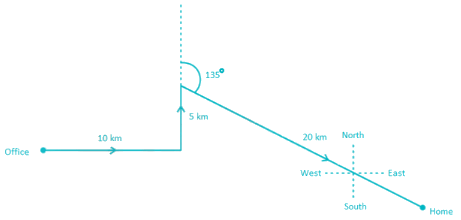Harish’s Home is clearly, in South – East Direction with respect to his office.

RRB NTPC Mock Test - 12 (English) - Question 50

Dactylogram is a term related to which of the following?

Detailed Solution for RRB NTPC Mock Test - 12 (English) - Question 50
• Dactylogram is the name given to the fingerprint of a person.
• It is a person's indelible mark.
• In today’s world, various mobile uses the Touch ID and dactylogram sensor to secure electronic devices.
RRB NTPC Mock Test - 12 (English) - Question 51

The definition for electric flux is ________.

Detailed Solution for RRB NTPC Mock Test - 12 (English) - Question 51
• Electric flux can be defined as the measure of the distribution of the electric field through a given surface. But, electric field in itself cannot flow.
• It is a way of describing the electric field strength at any distance from the charge causing the field.
RRB NTPC Mock Test - 12 (English) - Question 52

Some equations have been solved on the basis of a certain pattern. Find the correct answer for the unsolved equation on that basis.
If (a\$ b) = 3b – 2a, × = (4 \$ 3), then find out (X \$ (13 \$ 12)?

Detailed Solution for RRB NTPC Mock Test - 12 (English) - Question 52

Given in the question that (a \$ b) = 3b -2a.

X = (4 \$ 3) = (3 × 3 – 2 × 4) = 1

(13 \$ 12) = (3 × 12 – 2 × 13) = 10

(X \$ (13 \$ 12) = (1 \$ 10) = (3 × 10 – 2 × 1) = 28

So, 28 is the answer.

RRB NTPC Mock Test - 12 (English) - Question 53

Which among the following are also known as ‘Mountains of Accumulation’?

Detailed Solution for RRB NTPC Mock Test - 12 (English) - Question 53
• Volcanic Mountains is also known as Mountain of Accumulation
• They are formed due to the accumulation of volcanic material.
• Examples: Mt Fuji in Japan, Cotopaxi in the Andes, Mt. Mayon in the Philippines, etc.
RRB NTPC Mock Test - 12 (English) - Question 54

The calendar of 1999 is similar to which of the following years?

Detailed Solution for RRB NTPC Mock Test - 12 (English) - Question 54

Number of odd days in 1999 = 1

Number of odd days from 1999 till 2000 = 1 + 2 = 3 odd days

Number of odd days from 1999 till 2001 = 3 + 1 = 4 odd days

Number of odd days from 1999 till 2002 = 4 + 1 = 5 odd days

Number of odd days from 1999 till 2003 = 5 + 1 = 6 odd days

Number of odd days from 1999 till 2004 = 6 + 2 = 8 odd days

Number of odd days from 1999 till 2005 = 8 + 1 = 9 odd days

Number of odd days from 1999 till 2006 = 9 + 1 = 10 odd days

Number of odd days from 1999 till 2007 = 10 + 1 = 11 odd days

Number of odd days from 1999 till 2008 = 11 + 2 = 13 odd days

Number of odd days from 1999 till 2009 = 13 + 1 = 14 odd days = 0 odd days

Hence, calendar of 1999 is similar to that of 2010.

RRB NTPC Mock Test - 12 (English) - Question 55

The product of two numbers is y/x. If one of the numbers is x/y2, then the other number is

Detailed Solution for RRB NTPC Mock Test - 12 (English) - Question 55

Given,

Let other number be M.

Then,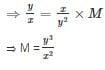RRB NTPC Mock Test - 12 (English) - Question 56

If (a + b)– 2(a + b) = 80 and ab = 16, then what can be the value of 3a – 19b?

Detailed Solution for RRB NTPC Mock Test - 12 (English) - Question 56

(a + b)– 2(a + b) = 80

⇒ (a + b)– 2(a + b) + 1 = 81

⇒ (a + b – 1)2 = 81

⇒ a + b – 1 = 9

⇒ a + b = 10

given ab = 16

⇒ a = 8 and b = 2

⇒ 3a – 19b = 24 – 38 = -14

RRB NTPC Mock Test - 12 (English) - Question 57

Which of the following jumble word is not a name of any Author?

Detailed Solution for RRB NTPC Mock Test - 12 (English) - Question 57

1) YVAS DEV = VED VYAS

2) CLESHAR DKNIC​E = CHARLES DICKEN

3) KJ OWLRNIG = JK ROWLING

4) HINSAC TRNELDUKA = SACHIN TENDULKAR (NOT A NAME OF ANY AUTHOR)

Hence, HINSAC TRNELDUKA is the correct answer.

RRB NTPC Mock Test - 12 (English) - Question 58

A merchant purchases a watch for Rs 510 and fixes its marked price in such a way that after allowing a discount of 15%, he earns a profit of 30%. What is the marked price (in Rs) of the watch?

Detailed Solution for RRB NTPC Mock Test - 12 (English) - Question 58

CP of watch = 510

Profit = 30%

⇒ SP = 510 + 0.3 × 510 = 663

Let the MP be x

⇒ SP = x - 0.15x = 0.85x

⇒ 0.85x = 663

⇒ x = 663/0.85 = Rs 780

RRB NTPC Mock Test - 12 (English) - Question 59

Which among the following is the national anthem of Spain?

Detailed Solution for RRB NTPC Mock Test - 12 (English) - Question 59
• The "Marcha Real" is the national anthem of Spain.
• "Jana Gana Mana" is the national anthem of India.
• "La Marseillaise" is the national anthem of France.
• "The Star-Spangled Banner" is the national anthem of the United States.
RRB NTPC Mock Test - 12 (English) - Question 60

The citizenship is mentioned in which of the following part of the Indian constitution?

Detailed Solution for RRB NTPC Mock Test - 12 (English) - Question 60
• Part II of the Constitution of India (Articles 5-11) deals with the Citizenship of India.

Citizenship of India can be acquired by the following ways:

• Citizenship at the commencement of the constitution of India
• Citizenship by birth
• Citizenship by descent
• Citizenship by registration
• Citizenship by naturalization
• Citizenship Amendment Bill 2016
RRB NTPC Mock Test - 12 (English) - Question 61

In the following question, from the given alternative words, select the word which CAN be formed using the letters of the given word.

BRYOPHYLLUM

Detailed Solution for RRB NTPC Mock Test - 12 (English) - Question 61

1) Burst → BRYOPHYLLUM, hence word cannot be formed (Letter S and T are not present)

2) Humble → BRYOPHYLLUM, hence word cannot be formed (Letter E is not present)

3) Hours → BRYOPHYLLUM, hence word cannot be formed (Letter S is not present)

4) Humbly → BRYOPHYLLUM, hence word can be formed.

Hence, ‘Humbly’ is the correct alternative.

RRB NTPC Mock Test - 12 (English) - Question 62

Which of the following straits separate Russia and Alaska?

Detailed Solution for RRB NTPC Mock Test - 12 (English) - Question 62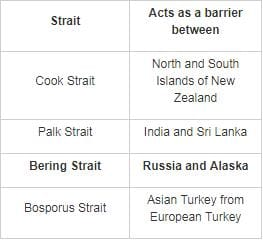RRB NTPC Mock Test - 12 (English) - Question 63

In the question below is given a statement followed by two conclusions numbered I and II. You have to assume everything in the statement to be true, then consider the two conclusions together and decide which of them logically follow beyond a reasonable doubt from the information given in the statement. Give answer.

Statement:
Death keeps no calendar.

Conclusions:
I. You can die only at night.
II. Everyone has to die one day.

Detailed Solution for RRB NTPC Mock Test - 12 (English) - Question 63

The first conclusion follows the given statement as death can come anytime but from the given statement, we cannot conclude that one can only die in the night. Hence, only conclusion II follows.

RRB NTPC Mock Test - 12 (English) - Question 64

In which of the following years Spinning Frame was invented?

Detailed Solution for RRB NTPC Mock Test - 12 (English) - Question 64
• Spinning Frame was invented in 1769 in England.
• Sir Richard Arkwright was the inventor of Spinning Frame
• It is used for spinning thread or yarn from fibers such as wool or cotton in a mechanized way.
RRB NTPC Mock Test - 12 (English) - Question 65

Present age of Ashok is twice the present age of Kishan. After 7 years the Kishan’s age will be 4 times of the Kartik’s present age. If Kartik celebrated his 9th birthday 3 years ago, then what will be the present age (in years) of Ashok?

Detailed Solution for RRB NTPC Mock Test - 12 (English) - Question 65

1) Kartik celebrate his 9th birthday 3 years ago,

Present age of Kartik = 9 + 3 = 12 years

2) After 7 years the Kishan age will be 4 times of Kartik age

7 + Kishan present age = 4 (Kartik present age)

Kishan present age = (4 × 12) - 7

48 - 7 = 41 years.

3) The present age of Ashok is twice the present age of Kishan.

Present age of Ashok = 2 × (Present age of Kishan)

= 2 × 41 = 82 years.

Hence 82 is the correct answer.

RRB NTPC Mock Test - 12 (English) - Question 66

A car can cover a certain distance in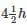If the speed is increased by 10 km/h, it would take 1/2 h less to cover the same distance. Find the slower speed of the car.

Detailed Solution for RRB NTPC Mock Test - 12 (English) - Question 66

Ratio of time :

= 9/2 : (9/2 – ½)

= 9/2 : 4

= 9 : 8

Speed of car increased by 10km/h

Then,

∴ Slower speed of car = 8 × 10 = 80km/h

RRB NTPC Mock Test - 12 (English) - Question 67

In each question below is given one statement that is followed by two assumptions numbered I and II. Consider the statement and decide which of the given assumptions is implicit.

Statement: Honest people do not prefer to work in government sector unless they want to change the system.

Assumption I: The government system is flawed with corruption.
Assumption II: Given that one knows about corruption in the public sector, people who wish to work in such sector are greedy and not honest.

Detailed Solution for RRB NTPC Mock Test - 12 (English) - Question 67

The only reason why honest people won't work in a system is if the system is not run with honesty hence assumption I is implicit. Every person working in the public sector cannot be dishonest as there may be people who work hard to bring change in the system with their honesty. Hence assumption II is not implicit. Only assumption I is implicit.

RRB NTPC Mock Test - 12 (English) - Question 68

If 200 meters long train takes 40 seconds to cross a pole, then what is the speed (in km/h) of the train?

Detailed Solution for RRB NTPC Mock Test - 12 (English) - Question 68

Speed = Distance covered/Time required

To cross a pole, the train has to cover a distance equal to its length.

∴ Distance covered = 200 m

Time taken = 40 seconds

∴ Speed = 200m/40s = (200/1000)/(40/3600) = 18 km/h

RRB NTPC Mock Test - 12 (English) - Question 69

Which of the following soil is also known as Omnibus soil?

Detailed Solution for RRB NTPC Mock Test - 12 (English) - Question 69
• Red Soil is also known as Omnibus soil.
• It is red in colour because of Ferric oxide.
• It is found in eastern and southern part of the Deccan plateau, Orissa, Chhattisgarh and southern parts of the middle Ganga plain.
• The main crops cultivated in red soil are wheat, cotton, pulses, tobacco, oilseeds, potato etc.
RRB NTPC Mock Test - 12 (English) - Question 70

Oxygen is transported in blood mainly by _____.

Detailed Solution for RRB NTPC Mock Test - 12 (English) - Question 70

The red blood cells are also called erythrocytes. The red blood cells contain an iron-containing pigment called hemoglobin which binds to oxygen.

RRB NTPC Mock Test - 12 (English) - Question 71

Total cost of 50 tables is Rs. 20000. If cost of each table increases by 30% and number of tables decrease by 20%, then what will be the total cost (in Rs.) of tables?

Detailed Solution for RRB NTPC Mock Test - 12 (English) - Question 71

Cost of 50 tables = Rs. 20000

∴ Cost of each table = Rs. (20000/50) = Rs. 400

After 30% increase,

Cost of each table = Rs. (400 + 400 × 30/100) = Rs. 520

After 20% decrease, no. of tables = 50 - 50 × 20/100 = 40

∴ Total cost,

⇒ 40 × 520

⇒ Rs. 20800

RRB NTPC Mock Test - 12 (English) - Question 72

Tibetan Plateau is an example of which among the following plateaus?

Detailed Solution for RRB NTPC Mock Test - 12 (English) - Question 72
• Tibetan Plateau is example of Inter-mountainous Plateau.
• Inter-mountainous Plateau is formed between mountains.
• Tibetan Plateau is called the "Roof of the World".
• It is also known as Qinghai-Tibet.
• Generally, the height of Plateau ranges from 300 to 500 feet but the height of Tibetan Plateau is 16,000 ft.
RRB NTPC Mock Test - 12 (English) - Question 73

How much will be the compound interest to be paid on a principal amount of Rs. 53,000 after 2 years at the rate of 4% p.a.?

Detailed Solution for RRB NTPC Mock Test - 12 (English) - Question 73

Amount received when interest is compounded = P[1 + {r/(n × 100)}]n×t

Where, P is the principal amount

r Is the rate of interest per annum

n is the number of compoundings in a year

t is the time period in years

Here, P = 53000, r = 4%, t = 2 years, n = 1

Amount = 53000 [1 + {4/(1 × 100)}]1×2

Amount = 57324.8

Amount is nothing but the summation of principal and interest

⇒ Principal + Interest = 57324.8

⇒ Interest = 57324.8 – 53000

∴ Interest = Rs. 4324.8

RRB NTPC Mock Test - 12 (English) - Question 74

Who among the following is the author of the book “The Sher Shah of Kargil: Captain Vikram Batra”?

Detailed Solution for RRB NTPC Mock Test - 12 (English) - Question 74
• The author of the book “The Sher Shah of Kargil: Captain Vikram Batra” is Deepak Surana.
• It was launched in Bangalore on September 10, 2017.
• It is the biography of Kargil war hero Late Captain Vikram Batra.
• It is a story of the young captain, which takes you through the various dimensions of Captain Batra’s life.
RRB NTPC Mock Test - 12 (English) - Question 75

Who among the following won 2018 Asian Player of the year award in hockey?

Detailed Solution for RRB NTPC Mock Test - 12 (English) - Question 75
• Manpreet Singh has been honored with 2018 Asian Player of the year award.
• Manpreet Singh is an Indian field hockey player and the captain of India men's national field hockey team since May 2017.
• Manpreet received the Arjuna Award from President of India Ram Nath Kovind in August 2018.
RRB NTPC Mock Test - 12 (English) - Question 76

Identify the diagram that best represents the relationship among the given classes.
Sky, Continent, Ocean

Detailed Solution for RRB NTPC Mock Test - 12 (English) - Question 76

Sky, Continent and Ocean have no intersection between them, so the Venn diagram that represents their relationship is,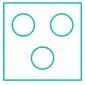Hence, option 2 is correct.

RRB NTPC Mock Test - 12 (English) - Question 77

What will come in place of question Mark ‘?’ in the following number question?

(756 + 62 × ?) ÷ 3 = 57 × 9 + 49

Detailed Solution for RRB NTPC Mock Test - 12 (English) - Question 77

Follow BODMAS rule to solve this question, as per the order given below,

Step-1- Parts of an equation enclosed in 'Brackets' must be solved first, and in the bracket,

Step-2- Any mathematical 'Of' or 'Exponent' must be solved next,

Step-3- Next, the parts of the equation that contain 'Division' and 'Multiplication' are calculated,

Step-4- Last but not least, the parts of the equation that contain 'Addition' and 'Subtraction' should be calculated.

Given expression is,

⇒ (756 + 62 × ?) ÷ 3 = 57 × 9 + 49

⇒ (756 + 62 × ?) ÷ 3 = 513 + 49

⇒ (756 + 62 × ?) ÷ 3 = 562

⇒ (756 + 62 × ?) = 562 × 3

⇒ (756 + 62 × ?) = 1686

⇒ 62 × ? = 1686 - 756

⇒ 62 × ? = 930

⇒ ? = 930 / 62

⇒ ? = 15

RRB NTPC Mock Test - 12 (English) - Question 78

Which among these glands is also known as the third eye in the human body?

Detailed Solution for RRB NTPC Mock Test - 12 (English) - Question 78
• Pineal Gland is also known as the third eye in the human body.
• It produces melatonin and serotonin-derived hormone.
• Serotonin-derived hormone controls waking up and sleeping.
• Melatonin-derived hormone controls the formation of sex hormone at the time of matting.
RRB NTPC Mock Test - 12 (English) - Question 79

Which of the following part of the Indian constitution deals with Election and Election commission?

Detailed Solution for RRB NTPC Mock Test - 12 (English) - Question 79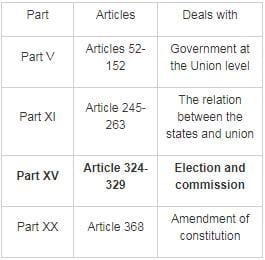RRB NTPC Mock Test - 12 (English) - Question 80

There are some pigeons and some tigers in a forest. If the total number of animal heads in the forest is 850 and the total number of animal legs is 1848, what is the number of pigeons in the forest?

Detailed Solution for RRB NTPC Mock Test - 12 (English) - Question 80

Let the number of pigeons and tigers be x and y respectively.

∴ according to the first given condition,

x + y = 850   ---(1)

A pigeon has 2 legs and a tiger has 4 legs

∴ According to the 2nd given condition,

2x + 4y = 1848  ---(2)

Solving the equations (1) and (2) simultaneously,

We get,

x = 776 and y = 74

∴ the number of pigeons in the forest is 776.

RRB NTPC Mock Test - 12 (English) - Question 81

From the given answer figures, select the one in which the question figure is hidden/embedded?Detailed Solution for RRB NTPC Mock Test - 12 (English) - Question 81

If we look closely, figure(X) is hidden in figure 2 as shown below.Hence, the answer should be figure 2.

RRB NTPC Mock Test - 12 (English) - Question 82

Select an appropriate figure from the four options that would complete the figure.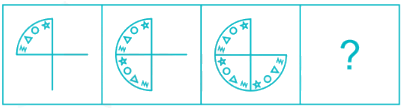Detailed Solution for RRB NTPC Mock Test - 12 (English) - Question 82

In fig.1 a curve is drawn. Within a curve a star, a circle, a triangle and a spiral symbol is positioned.

In fig.2 a curve is drawn. Within a curve a star, a circle, a triangle and a spiral symbol is positioned.

In fig.3 a curve is drawn. Within a curve a star, a circle, a triangle and a spiral symbol is positioned.

In fig.4 a curve is drawn. Within a curve a star, a circle, a triangle and a spiral symbol is positioned.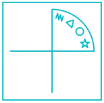Thus, in all the figures all the symbols are arranged in one order.

Hence, the answer is option 2.

RRB NTPC Mock Test - 12 (English) - Question 83

The ratio of two numbers is 5 ∶ 9 and their difference is 44. What will be the smaller number among both the numbers?

Detailed Solution for RRB NTPC Mock Test - 12 (English) - Question 83

Let two numbers be 5a and 9a

According to question,

Difference = 44

⇒ 9a – 5a = 44

⇒ 4a = 44

⇒ a = 11

∴ Smallest number = 5a = 5 × 11 = 55

RRB NTPC Mock Test - 12 (English) - Question 84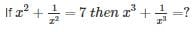Detailed Solution for RRB NTPC Mock Test - 12 (English) - Question 84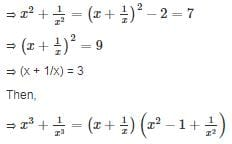= 3 × (7 – 1)

= 18

RRB NTPC Mock Test - 12 (English) - Question 85

In the given figure, how many black buttons are shirts?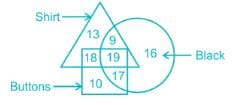Detailed Solution for RRB NTPC Mock Test - 12 (English) - Question 85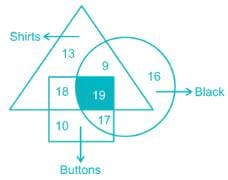Clearly, 19 black buttons are shirts

RRB NTPC Mock Test - 12 (English) - Question 86

Tina and Sujata were roaming in a shopping mall. Pointing to a lady in the food court, Tina said, “Her father is the son of my only paternal aunt’s father. My grandfather has only one son and one daughter”. How is lady related to that Tina?

Detailed Solution for RRB NTPC Mock Test - 12 (English) - Question 86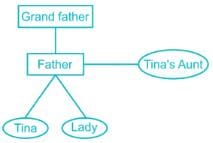We can see that Lady is sister of Tina.

RRB NTPC Mock Test - 12 (English) - Question 87

14 years ago, Mia’s grandmother was 6 times older than Mia. She would be 3.75 times of Mia’s age 4 years from now. 6 years ago, what was the ratio of Mia’s age to that of her grandmother?

Detailed Solution for RRB NTPC Mock Test - 12 (English) - Question 87

Let the present age of Mia be X and grandmother’s age be Y.

Condition(i) 1 Age of Mia and Grandmother 14 years ago

Y - 14 = 6(X – 14)

⇒ Y – 14 = 6X – 84

⇒ 6X – Y = 70      ----(i)

Condition (ii) Age of Mia and Grandmother after 4 years from present age

Y + 4 = 3.75(X + 4)

⇒ 15X – 4Y = -44      ----(ii)

Solving (i) and (ii)

X = 36; Y = 146

∴ Ratio of Mia’s age to that of her grandmother 6 years ago = (36 - 6) : (146 - 6) = 3 : 14

RRB NTPC Mock Test - 12 (English) - Question 88

FTP used in computer technology stands for which of the following?

Detailed Solution for RRB NTPC Mock Test - 12 (English) - Question 88
• FTP stands for File Transfer Protocol.
• It is a standard network protocol used to transfer files between computers over a TCP/IP network.
• Every FTP has a specific FTP address that is used to transfer the files to the internet.
• You will need that address, username & password to access the files on the Web server.
RRB NTPC Mock Test - 12 (English) - Question 89

In which year an amendment was made for Panchayati Raj to receive Constitutional status?

Detailed Solution for RRB NTPC Mock Test - 12 (English) - Question 89
• In 1992 an amendment made in which Panchayati Raj received Constitutional Status.
• Panchayati Raj refers to the system of local self-government.
• It was formalized by the 73rd amendment to the Indian Constitution.
RRB NTPC Mock Test - 12 (English) - Question 90

Three numbers A, B and C are in the ratio of 10 : 12 : 23. If sum of these numbers is 540, ratio between the difference of B and A and the difference of C and B is-

Detailed Solution for RRB NTPC Mock Test - 12 (English) - Question 90

Let the value of A be 10x, then value of B and C will be 12x and 23x respectively.

Sum of the numbers = 540

⇒ 10x + 12x + 23x = 540

⇒ 45x = 540

⇒ x = 12

∴ A = 10x = 10 × 12 = 120

B = 12x = 12 × 12 = 144

And C = 23x = 23 × 12 = 276

Difference between B and A = 144 – 120 = 24

Difference between C and B = 276 – 144 = 132

∴ Required ratio = 24 ∶ 132 = 2 ∶ 11

RRB NTPC Mock Test - 12 (English) - Question 91

In the question, two statements are given, followed by two conclusions, I and II. You have to consider the statements to be true even if it seems to be at variance from commonly known facts. You have to decide which of the given conclusions, if any, follows from the given statements.

Statement I: No rings are bangles
Statement II: All gold are rings

Conclusion I: No bangles are gold
Conclusion II: Some rings are gold

Detailed Solution for RRB NTPC Mock Test - 12 (English) - Question 91

The least possible diagram of this question is as follows,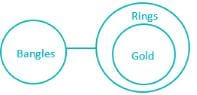Conclusion I: No bangles are gold →True.
Conclusion II: Some rings are gold →True.

Hence, both conclusions I and II follow.

RRB NTPC Mock Test - 12 (English) - Question 92

Who among the following won the Battle of Kanauj?

Detailed Solution for RRB NTPC Mock Test - 12 (English) - Question 92
• Shershah won the Battle of Kanauj on 17th, May 1540.
• The war fought between Sher Shah and Humayun.
• After the victory of Shershah, Agra was occupied by him
RRB NTPC Mock Test - 12 (English) - Question 93

In which of the following term one-year plans were created instead of five-year plans?

Detailed Solution for RRB NTPC Mock Test - 12 (English) - Question 93
• Due to the volatile political situations, for the year 1990-1991 and 1991-1992 annual plans were created.
• The Eighth Plan was to begin from April 1990 but successive governments were unable to finalize the plan document.
• The 8th Plan was finally launched in April 1992.
• In the year 1991, India faced a crisis in foreign exchange (forex) reserves.
• To overcome these crises, India launched LPG (Liberalisation, Privatisation, and Globalisation).
RRB NTPC Mock Test - 12 (English) - Question 94

Study the following graph carefully and answer the questions.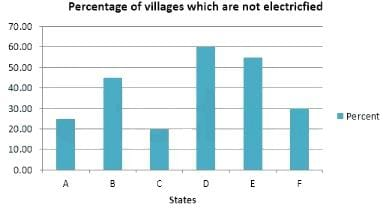Q. Which state has twice the percentage of villages electrified in comparison to state D?

Detailed Solution for RRB NTPC Mock Test - 12 (English) - Question 94

From the graph,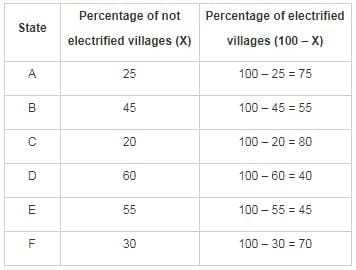∴ We can clearly observe that state C has twice the percentage of villages electrified in comparison to state D.

RRB NTPC Mock Test - 12 (English) - Question 95

Direction: Study the given information carefully and answer the question that follows.
Eleven students P, B, C, Q, E, F, R, H, I, S and T are sitting in the first row of the class facing the Teacher.

1. Q who is to the immediate left of F is third to the right of C.
2. P is second to the right of E, who is at one of the ends.
3. S is the immediate neighbour of P and B and third to the left of R.
4. H is to the immediate left of Q and third to the Left of I.

Q. Which of the following groups of friends is sitting to the right of R?

Detailed Solution for RRB NTPC Mock Test - 12 (English) - Question 95

Eleven students P, B, C, Q, E, F, R, H, I, S and T are sitting in the first row of the class facing the teacher.

P is second to the right of E, who is at one of the ends. Clearly, E must be at left most to place P 2nd to the right of E.

S is the immediate neighbour of P and B and third to the left of R. So S must be between P and B to become the immediate neighbour of both.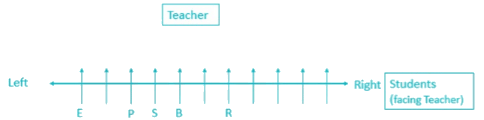H is to the immediate left of Q and third to the Left of I; this holds true only when H sits immediate right to R.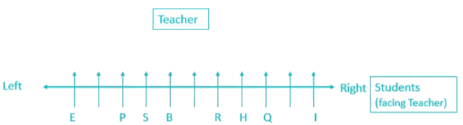Q who is to the immediate left of F is third to the right of C.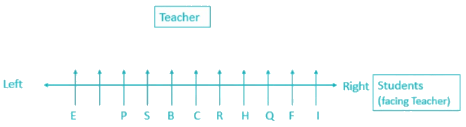Now, only T is left so it is automatically filled between E and P.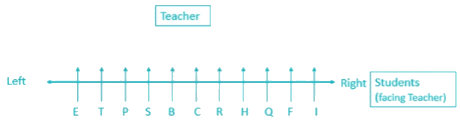Clearly, H, Q, F and I are sitting right to the R.

RRB NTPC Mock Test - 12 (English) - Question 96

Direction: Study the given information carefully and answer the question that follows.
Eleven students P, B, C, Q, E, F, R, H, I, S and T are sitting in the first row of the class facing the Teacher.

1. Q who is to the immediate left of F is third to the right of C.
2. P is second to the right of E, who is at one of the ends.
3. S is the immediate neighbour of P and B and third to the left of R.
4. H is to the immediate left of Q and third to the Left of I.

Q. Who is sitting in the middle of the row?

Detailed Solution for RRB NTPC Mock Test - 12 (English) - Question 96

Eleven students P, B, C, Q, E, F, R, H, I, S and T are sitting in the first row of the class facing the teacher.

P is second to the right of E, who is at one of the ends. Clearly, E must be at left most to place P 2nd to the right of E.

S is the immediate neighbour of P and B and third to the left of R. So S must be between P and B to become the immediate neighbour of both.H is to the immediate left of Q and third to the Left of I; this holds true only when H sits immediate right to R.Q who is to the immediate left of F is third to the right of C.Now, only T is left so it is automatically filled between E and P.Clearly, C sits in the middle of the row.

RRB NTPC Mock Test - 12 (English) - Question 97

Direction: Study the given information carefully and answer the question that follows.
Eleven students P, B, C, Q, E, F, R, H, I, S and T are sitting in the first row of the class facing the Teacher.

1. Q who is to the immediate left of F is third to the right of C.
2. P is second to the right of E, who is at one of the ends.
3. S is the immediate neighbour of P and B and third to the left of R.
4. H is to the immediate left of Q and third to the Left of I.

Q. Which of the following statements is true in the context of the above sitting arrangement?

Detailed Solution for RRB NTPC Mock Test - 12 (English) - Question 97

Eleven students P, B, C, Q, E, F, R, H, I, S and T are sitting in the first row of the class facing the teacher.

P is second to the right of E, who is at one of the ends. Clearly, E must be at left most to place P 2nd to the right of E.

S is the immediate neighbour of P and B and third to the left of R. So S must be between P and B to become the immediate neighbour of both.H is to the immediate left of Q and third to the Left of I; this holds true only when H sits immediate right to R.Q who is to the immediate left of F is third to the right of C.Now, only T is left so it is automatically filled between E and P.Clearly, B is sitting between S and C is true.

RRB NTPC Mock Test - 12 (English) - Question 98

In a certain code language ‘FIGHTER’ is written as ‘@SR^*B&’ and ‘CHAIR’ is written as ‘V^WS&’. How is ‘PICTURE’can be written in that code?

Detailed Solution for RRB NTPC Mock Test - 12 (English) - Question 98

The codes have been assigned as follows,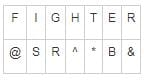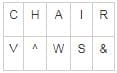Similarly,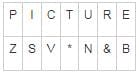RRB NTPC Mock Test - 12 (English) - Question 99

In certain coding language, ‘pan’ en san' means 'sky is blue’, 'han fan en' means 'rose is red', 'han en tan' means ‘red’ is copy.

How is "blue rose is copy" written in that code?

Detailed Solution for RRB NTPC Mock Test - 12 (English) - Question 99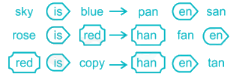Therefore,

'is' → 'en'

Rose → fan

Copy → tan

Blue → pan/san

Hence, the most suitable code should be 'fan san tan en'.

RRB NTPC Mock Test - 12 (English) - Question 100

In the following question, select the related image from the given alternatives.Detailed Solution for RRB NTPC Mock Test - 12 (English) - Question 100

The pattern followed here is:Hence, the answer is option 1.

## RRB NTPC Mock Tests Series

20 tests
 Use Code STAYHOME200 and get INR 200 additional OFF Use Coupon Code
Information about RRB NTPC Mock Test - 12 (English) Page
In this test you can find the Exam questions for RRB NTPC Mock Test - 12 (English) solved & explained in the simplest way possible. Besides giving Questions and answers for RRB NTPC Mock Test - 12 (English), EduRev gives you an ample number of Online tests for practice

20 tests